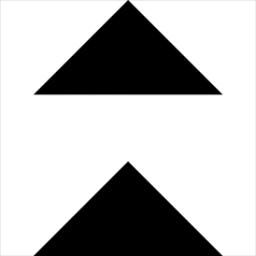# Area of RT 2

Calculate the area of right triangle whose legs have a length 5.8 cm and 5.8 cm.

Correct result:

S =  16.82 cm2

#### Solution:We would be pleased if you find an error in the word problem, spelling mistakes, or inaccuracies and send it to us. Thank you!Tips to related online calculators

#### You need to know the following knowledge to solve this word math problem:

We encourage you to watch this tutorial video on this math problem:

## Next similar math problems:

• Here isHere is a data set (n=117) that has been sorted. 10.4 12.2 14.3 15.3 17.1 17.8 18 18.6 19.1 19.9 19.9 20.3 20.6 20.7 20.7 21.2 21.3 22 22.1 22.3 22.8 23 23 23.1 23.5 24.1 24.1 24.4 24.5 24.8 24.9 25.4 25.4 25.5 25.7 25.9 26 26.1 26.2 26.7 26.8 27.5 27.6 2
• Four prismsQuestion No. 1: The prism has the dimensions a = 2.5 cm, b = 100 mm, c = 12 cm. What is its volume? a) 3000 cm2 b) 300 cm2 c) 3000 cm3 d) 300 cm3 Question No.2: The base of the prism is a rhombus with a side length of 30 cm and a height of 27 cm. The heig
• IS trapezoidCalculate the length of diagonal u and height v of isosceles trapezoid ABCD, whose bases have lengths a = |AB| = 37 cm, c = |CD| = 29 cm and legs b = d = |BC| = |AD| = 28 cm.
• Chocolate rollThe cube of 5 cm chocolate roll weighs 30 g. How many calories will contain the same chocolate roller of a prism shape with a length of 0.5 m whose cross section is an isosceles trapezoid with bases 25 and 13 cm and legs 10 cm. You know that 100 g of this
• Diagonalhe rectangular ABCD trapeze, whose AD arm is perpendicular to the AB and CD bases, has area 15cm square. Bases have lengths AB = 6cm, CD = 4cm. Calculate the length of the AC diagonal.
• Truncated pyramidThe concrete pedestal in the shape of a regular quadrilateral truncated pyramid has a height of 12 cm, the pedestal edges have lengths of 2.4 and 1.6 dm. Calculate the surface of the base.
• Right triangle ABCCalculate the perimeter and area of a right triangle ABC, if you know the length of legs 4 cm 5.5 cm and 6.8 cm is hypotenuse.
• Area 4gonCalculate area of 4-gon, two and the two sides are equal and parallel with lengths 11, 5, 11 and 5. Inner angles are 45°, 135°,45°, 135°.
• Rectangular trianglesThe lengths of corresponding sides of two rectangular triangles are in the ratio 2:5. At what ratio are medians relevant to hypotenuse these right triangles? At what ratio are the contents of these triangles? Smaller rectangular triangle has legs 6 and 8
• Isosceles trapezoidThe lengths of the bases of the isosceles trapezoid are in the ratio 5:3, the arms have a length of 5 cm and height = 4.8 cm. Calculate the circumference and area of a trapezoid.
• Right triangleRight triangle legs has lengths 630 mm and 411 dm. Calculate the area of this triangle.
• SimilarityAre two right triangles similar to each other if the first one has a acute angle 70° and second one has acute angle 20°?
• Reverse Pythagorean theoremGiven are lengths of the sides of the triangles. Decide which one is rectangular: Δ ABC: 77 dm, 85 dm, 36 dm ? Δ DEF: 55 dm, 82 dm, 61 dm ? Δ GHI: 24 mm, 25 mm, 7 mm ? Δ JKL: 32 dm, 51 dm, 82 dm ? Δ MNO: 51 dm, 45 dm, 24 dm ?
• Roof coverAbove the pavilion with a square ground plan with a side length of a = 12 m is a pyramid-shaped roof with a height v = 4.5 m. Calculate how much m2 of sheet metal is needed to cover this roof if 5.5% of the sheet we must add for joints and waste.
• The basesThe bases of the isosceles trapezoid ABCD have lengths of 10 cm and 6 cm. Its arms form an angle α = 50˚ with a longer base. Calculate the circumference and content of the ABCD trapezoid.
• Chimney and treeCalculate the height of the factory chimney, which casts a shadow 6.5 m long in the afternoon. At the same time, a 6 m high tree standing near it casts a shadow 25 dm long.
• KiteJohn a kite, which is diamond shaped. Its diagonals are 60 cm long and 90 cm long. Calculate: a) the diamond side b) how much paper John needs to make a kite if he needs paper on both sides and needs 5% of the paper for bending.